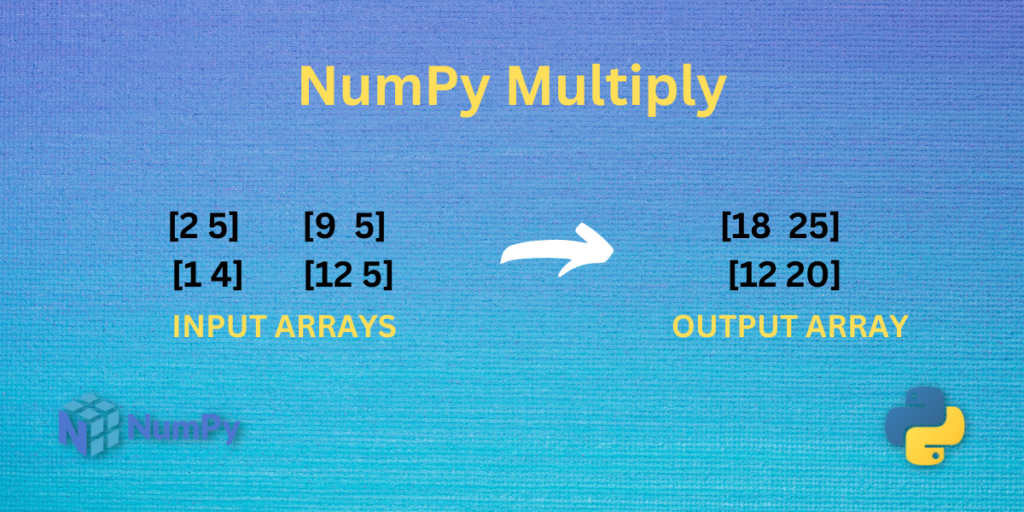# NumPy multiply – Illustrated in a Simple WayHey everyone! Welcome to another tutorial on NumPy functions. In this tutorial, we will explore the NumPy multiply function in detail.

We all do multiplication operations in our daily life. Be it our mathematics class or the calculations done by the shopkeeper to keep track of the items in the shop. Today we will see how can we multiply two numbers or two arrays of numbers using python programming.

Let’s get started.

Also read: NumPy linalg.det – Compute the determinant of the given array

## What is NumPy multiply?

NumPy multiply is one of the mathematical functions of the NumPy library that multiplies the inputs passed to the function.

Let’s take a look at the syntax of the function.

### Syntax of NumPy multiply

```numpy.multiply(x1 , x2)
```

Here, the inputs `x1` and `x2` can be scalar numbers as well as a NumPy array of numbers.

## Working with NumPy multiply

Let’s do some python programming.

### NumPy multiply with Scalar Values

```# Importing the NumPy module
import numpy as np

a = 5
b = 4

print("The product of 5 and 4 is:",np.multiply(a , b))
```

Output

```The product of 5 and 4 is: 20
```

We first import the NumPy library using the `import` statement in the above snippet. In the `print` statement, the function `np.multiply(a,b)` is called where a and b are passed as input to the function.

In this snippet, the output is also a scalar value.

### NumPy multiply with a NumPy array and a Scalar value

```# Importing the NumPy module
import numpy as np

# Creating the  2-D array
a = np.array([[2 , 4 , 7] , [5 , 10 , 15]])

b = 10

c = np.multiply(a , b)

print("Input Array:\n",a)
print("After multiplying 10 to each value of the Input Array:\n",c)
```

Output

```Input Array:
[[ 2  4  7]
[ 5 10 15]]
After multiplying 10 to each value of the Input Array:
[[ 20  40  70]
[ 50 100 150]]
```

In the above example, a 2-D array of size 2×3 is created using the function `np.array()`. In the following lines, the function `np.multiply(a,b)` is called by passing `a` and `b` as arguments to the function where `a` is the NumPy array and `b` holds the scalar value of 10.

In the output, the function `np.multiply(a,b)` multiplied all the values of the NumPy array by 10.

### NumPy multiply with two Same- Sized NumPy arrays

```import numpy as np

# Creating 2x2 array
a = np.array([[2 , 5] , [1 , 4]])

b = np.array([[9 , 5] , [21 , 34]])

# Using the multiply function
c = np.multiply(a , b)

# Printing the values
print("Array 1:\n",a)
print("Array 2:\n",b)

print("Output array:\n",c)
```

Output

```Array 1:
[[2 5]
[1 4]]
Array 2:
[[ 9  5]
[21 34]]
Output array:
[[ 18  25]
[ 21 136]]
```

In this example, two NumPy arrays of size 2×2 are created using the function `np.array()` and are stored in the variables `a` and `b`. Next, the function `np.multiply(a,b)` is called by passing `a` and `b` as arguments where `a` and `b` are the NumPy arrays that we created previously using the function `np.array()`.

In the output, the array contains the product of the values at the same position in the two input arrays.

Note: The output array has the same size as the input array.

### NumPy multiply with a Matrix and a Vector

```import numpy as np

# Creating a vector or 1-D array
a = np.array((10 , 20 , 30))

# Creating a matrix or 2-D array
b = np.array([[1 , 2 , 4] , [8 , 10 , 16]])

c = np.multiply(a , b)

print("Array 1:\n",a)
print("Array 2:\n",b)

print("Output Array:\n",c)
```

Output

```Array 1:
[10 20 30]
Array 2:
[[ 1  2  4]
[ 8 10 16]]
Output Array:
[[ 10  40 120]
[ 80 200 480]]
```

This is the most interesting example. Here, we created a vector or a 1-D array having 3 elements and a 2-D array or matrix of size 2×3 i.e. having 2 rows and 3 columns. In the following lines, the function `np.multiply(a,b)` is called by passing `a` and `b` as arguments where a is the vector and b is the matrix.

In this situation, NumPy performs broadcasting. It takes the vector and multiplies it with each row in the matrix. This is possible because the vector has the same number of elements as the number of columns in the matrix.

In the output array, the first row of elements is obtained by multiplying the vector with the first row of the matrix, and the second row of elements is obtained by multiplying the vector with the second row of the matrix.

With that, we completed all the examples for this tutorial. You should try using the function with examples of your choice and observe the outputs.

## Summary

In this article, we learned about the NumPy multiply function and practiced different types of examples. This is really a simple function to use and easy to understand. Keep exploring more such tutorials here.

## Reference

NumPy Documentation – NumPy multiply